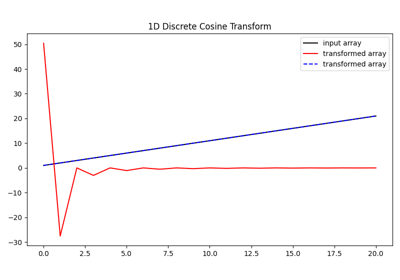# pylops.signalprocessing.DCT#

class pylops.signalprocessing.DCT(dims, type=2, axes=None, dtype='float64', workers=None, name='C')[source]#

Discrete Cosine Transform.

Apply 1D or ND-Cosine Transform along one or more axes of a multi-dimensional array of size dims.

This operator is an overload of scipy.fft.dctn in forward mode and scipy.fft.idctn in adjoint mode.

Parameters
dims

Number of samples for each dimension

typeint, optional

Type of DCT (see scipy’s documentation for more details). Default type is 2.

axesint or list, optional

Axes over which the DCT is computed. If None, the transform is applied over all axes.

workers :obj:int, optional

Maximum number of workers to use for parallel computation. If negative, the value wraps around from os.cpu_count().

dtypeDTypeLike, optional

Type of elements in input array.

namestr, optional

Name of operator (to be used by pylops.utils.describe.describe)

Raises
ValueError

If type is different from 1, 2, 3, or 4.

Notes

The DCT operator applies the Discrete Cosine Transform in forward mode and the Inverse Discrete Cosine Transform in adjoint mode. This transform expresses a signal as a sum of cosine functions oscillating at different frequencies. By doing so, no information is lost and the energy is compacted into the top left corner of the transform. When applied to multi-dimensional arrays, the DCT operator is simply a cascade of one-dimensional DCT operators acting along the different axes,

Finally, note that the DCT operator is implemented with normalization mode norm="ortho" to ensure symmetric scaling.

Attributes
shapetuple

Operator shape

explicitbool

Operator contains a matrix that can be solved explicitly (True) or not (False)

Methods

 __init__(dims[, type, axes, dtype, workers, ...]) adjoint() apply_columns(cols) Apply subset of columns of operator cond([uselobpcg]) Condition number of linear operator. conj() Complex conjugate operator div(y[, niter, densesolver]) Solve the linear problem $$\mathbf{y}=\mathbf{A}\mathbf{x}$$. dot(x) Matrix-matrix or matrix-vector multiplication. eigs([neigs, symmetric, niter, uselobpcg]) Most significant eigenvalues of linear operator. matmat(X) Matrix-matrix multiplication. matvec(x) Matrix-vector multiplication. reset_count() Reset counters rmatmat(X) Matrix-matrix multiplication. rmatvec(x) Adjoint matrix-vector multiplication. todense([backend]) Return dense matrix. toimag([forw, adj]) Imag operator toreal([forw, adj]) Real operator tosparse() Return sparse matrix. trace([neval, method, backend]) Trace of linear operator. transpose()

## Examples using pylops.signalprocessing.DCT#Discrete Cosine Transform

Discrete Cosine Transform